# Simulation numérique de la récupération de polluants en sous-sol

Heavy Oil Recovery SimulationKHELIFA Mansour  with Monsieur Pierre Horgue

ENSEEIHT Fluid Mechanics Department and IMFT

2012-2013

# Introduction

The Heavy Oil Recovery Simulation Project was made by Monsieur Mansour KHELIFA an ENSEEIHT student  from Fluid Mechanics department.  During the project, the student was supervised by Monsieur Pierre Horgue a researcher from the IMFT (Institut de Mécanique des Fluides de Toulouse).

The aim of this project is the development of a multiphase model in order to simulate the oil recovery process. The oil present in the underground comes from a leakage on an industrial site. The presented model is developed using the OpenFOAM platform.

From scientific point of view, this kind of problem is complex, mainly due to the presence of multiphase flow in the domain.

First of all we will study the problem in simple cases (one phase flow). After the completion of this work, we will deal with more complex situations (such as two-phase flow...).During all the project, the geometry considered will be like the geometry presented above : a square in which is introduced a tube corresponding to the producer well.

# Strategy and organization of the project

We decided to develop the two phase flow solver by starting with the development and the study of one phase flow solver.

First of all we  will integrated  in the one phase flow solver the permeability as a tensor and the gravity field.

To test this solver we will do several simulation  and by varying one parameter and fixing all the other parameters.

After  accomplishing the work on the one phase flow solver we will develop a two phase solver using a numerical method called IMPES (Implicit Pressure Explicit Saturation).

Moreover we will study how to reduce or delay the instabilities created by the method IMPES.

The code will be tested in several simulation without capillary pressure and for fluids of different viscosity and different rate flow of pumping.

Finally we will add the capillary pressure and test very simple correlations of capillary pressure.

# OpenFoam presentation

OpenFoam is a CFD open source code programmed in C++ language. It is mainly aimed to resolve partial derivative equations using finite volume method. OpenFoam is made up by toolboxes that are “easy” to program.
OpenFoam can use his integrated meshing software (blockMesh, snappyHexMesh
) or use meshing converter (Ansys, Salomé, ideas, CFX, Star-CD, Gambit, Gmsh...).

# Domain representation

The geometry chosen for the development of the method is a two-dimensional box in which there is one or several wells.

The wellbore influence is simply taken into account by adding a source term in the mass conservation equations. In order to have a flexibility in the positions of the wells, a constant, associated which this source term, is defined in the whole domain and must be equal to one where a wellbore is present or zero otherwise.

The source term must be negative as in the well the oil is extracted. We took a very simple model of well in order to implement the first simulations. For all the simulations in this project the source term will be equal to the local flow rate.

On the Top of the domain we defined a condition of constant pressure (Pref equal to the atmospheric pressure for example).On the rest of domain boundaries we have walls. Then we put a condition of zero velocity on those parts. When we take into account the gravity, the boundary condition on the pressure gradient on the bottom of the domain will be calculated as a function of the gravity field.# Permeability perturbation due to the well introduction

We know that the introduction of a pipe modifies the flow characteristics and change the local permeability. We can define an heterogeneous permeability. However in our case and as a first approach we will focus only on the permeability in the porous media. We will consider that the well introduction will not affect the permeability.

# Introduction and models used

In the real problem, there is the presence of three phases : air, oil and water. The study of multiphase flow is often complex. It's why, tackling directly the three phase-flow resolution could be very difficult and could lead to a misunderstanding of the simplest principles of the problem. Then  we decided, first of all to begin our study with simple cases and studying them for different parameters combinations.

In the one phase flow case we consider that there is only one fluid presents in the whole domain of resolution and we resolve the simple conservation equation in which Darcy law is injected.

We used the following equations :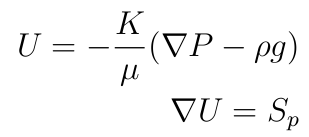where :

$U$ :  the velocity field

$P$ : the pressure

$S_p$ :  the source term

$K$ : the permeability

$g$ : the gravity

$\rho$ : the density

$\mu$ : the dynamic viscosity

In some studies the gravity will be neglected.

To resolve the conservation equation in which Darcy law is injected  we developed a solver that we called a Darcy solver or darcyFoam by modifying the existing laplacianFoam solver in OpenFoam solver directory. Then we modified it in order to compute the pressure field and having Darcy velocity.

After developing this simple Darcy solver, we added a source term that corresponds to the rate flow in the extractor well.

The second step was the definition of the permeability, no more as a simple scalar but as  a scalar field or as a tensor.

Finally we added to our solver the gravity, which  needed a real understanding of the gravity influence on boundaries conditions of the domain of study. Indeed the gravity introduction leads to a new boundary condition on the pressure gradient on the bottom of the domain and the inversion of the permeability tensor which is complex to program on OpenFoam. The boundary condition on the bottom will be expressed by :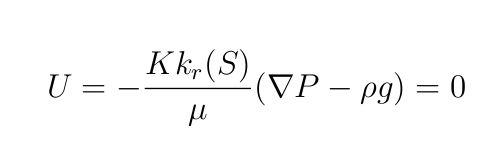On OpenFoam  we used flux through cells surface to set this boundary condition, then the condition of zero velocity on the domain bottom is transformed to a condition of zero flux.

The flux is expressed by :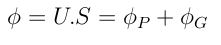S is the cell surface vector, where :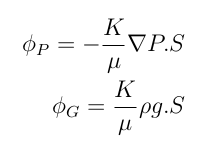Moreover $U=0$ leads to $\phi=0$,  then :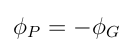Finally we have the following boundary condition on the pressure gradient :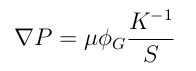To avoid having a matrix inversion, the permeability K was defined as a simple scalar or as a field of scalar as a first approach.

When the permeability is defined as a scalar or as a scalar field have :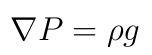In this parts we will study the influence of the anisotropy, the gravity and the flow rate on the flow to try different parameters and to study the stability of the solver.

In all the studies of this part, the fluid has the following physical properties :

$\rho=1000 \ Kg/m^3$

$\mu=6,4 \ 10^{-4} \ Kg/(m.s)$

Those properties are almost the properties of petrol.

Moreover the flow rate  called qwell will be fixed to :

$qwell=1 \ m^3/s$.

# Influence of the anisotropy

As we know the large majority of porous media that we can find in industry or in the nature present an anisotropy. It's why it is interesting to understand the influence of the anisotropy on the flow.

In order to take into account the anisotropy, we define the permeability as a tensor field in $m^2$:First of all we study a case with K defined as a tensor but without anisotropy in $m^2$:Then we can notice, that in the anisotropic K introduced, the permeability is more important in the horizontal direction than in the vertical or perpendicularly direction. Which means that we should have a more important velocity in the horizontal direction.

In the following study the gravity influence was neglected.

# Influence of the anisotropy on the velocity field

Velocity field in anisotropic case :Velocity field in isotropic case :We observe that in the anisotropic case the velocity magnitude is more important in the horizontal direction than in the vertical one as it was predicted in theory. Indeed this result is normal because the permeability is more important in the horizontal direction than in the other directions.

# Influence of the anisotropy on the pressure field

Pressure field in the case of isotropy :Pressure field in the case of anisotropy :As we have decreased the permeability in the vertical direction in the anisotropic case, the velocity is decreased which increases the pressure.

# Gravity influence

Taking into account the gravity in the darcyFoarm solver, is complex and needs a good basics in programming on OpenFoam.

Indeed having a gravity field involves a new boundary condition in the bottom of the domain on the pressure gradient.
Then the condition zero velocity on the bottom is expressed by :then we have the following boundary condition on the pressure gradient:After adding the gravity to the solver, we decided to compare the case with gravity to the case without gravity term that intervenes in Darcy law.
In this study the solver used is a solver without anisotropy, moreover the permeability will be equal to $K=7.10 {-7} \ m^2$.

Therefore we studied the influence of the gravity on the pressure and on the velocity fields :

# Gravity influence on the velocity field

Velocity field without gravity presence :Velocity field with gravity presence :We notice that the gravity has few impact on the velocity field.

# Gravity influence on the pressure field

Pressure without gravity field :Pressure with gravity field :We notice that the gravity decreases the negative maximum pressure.

# Well size influence

As on the field, a well implementation is hugely expensive and a mistake could lead to huge economical losses. It's important to know the best dimensions and the most adapted to the problem before drilling.

We tested in this part three well dimensions. And we studied the behavior of the flow as a function of the well diameter that we have called D.

The solver used is a solver without anisotropy, moreover the permeability will be equal to $K=7.10 {-7} \ m^2$.

# Influence of the well size on the velocity field

Velocity field D=0.2 mVelocity field D=0.5 mVelocicty field  D=1 mWe observe that an increasing in the well diameter  leads to a decreasing of the maximum velocity magnitud.

# Influence of the well size on the pressure field

Pressure field D=0.2 mPressure field D= 0.5 mPressure field D= 1 mWe observe that an increasing in the well diameter leads to a increasing of the maximum negative pressure.

# Influence of the flow rate

In this part, we will study the influence of the source term on the flow. The source term corresponds to the flow rate in the well. We call the flow rate qwell.

The study will be led with the parameters presented on the previous parts. The only parameters that will change is the flow rate. The solver used is a solver without anisotropy, moreover the permeability will be equal to $K=7.10 {-7} \ m^2$.

In some cases with very high rate flow the solutions obtained are not real because the Darcy low is valid only for small velocities.

# Influence of the flow rate on the velocity

Velocity field for the case : $qwell=0.5 \ m^3/s$Velocity field for the case : $qwell=1 \ m^3/s$Velocity field for the case : $qwell=10 \ m^3/s$An increasing of the flow rate induce an increasing in the velocity magnitude.

# Influence of the flow rate on the pressure field

Pressure field for the case : $qwell=0.5 \ m^3/s$Pressure field for the case : $qwell=1\ m^3/s$Pressure field for the case : $qwell=10 \ m^3/s$An increase in flow rate induce an increase of the negative pressure.

# Conclusion of monophase flow study

In conclusion, we succeeded to implement the gravity. The presence of the gravity leads to a real impacts on the flow.

It's important to take into account the anisotropy of the domain to have the best description of the flow.

However when we take into account the gravity the  definition of a boundary condition of zero velocity (zero flux in our solver) the bottom of the domain  leads to the matrix permeability inversion. The matrix inversion on OpenFoam is complex to implement. For this reason in the solver with gravity we defined the permeability as a scalar field or as a scalar.

The determination of the well size depends on the objectives of production fixed by the operator and the parameters of the problem.

Finally, our  simulations obtained by the solvers developed in this part are compatible with the theoretical studies found in the literature or data from real fields.

# Two phase flow

After the code validation in monophase flow case, we decided to add an additional phase to the solver. Then we developed a code that we called impesFoam. In the code, we resolved the conservation equations of the two phases to obtain the saturation and the the pressure field. We called the phase corresponding to air phase a and the phase that corresponds to the liquid (oil or water) phase b.

We used in all our studies the same geometry as the geometry that has been used in the previous parts which corresponds to a simple square, however the well size will be modified in certain cases.

First of all we resolved low and high values of saturation problems, after that we added to the developed solver the capillary pressure  and finally we did several simulations to study different regimes.

# Models used

In the  developed solver we resolve the  conservation equations to obtain the saturation field and the pressure field.

In general case of an isothermal flow we have to calculate in each point of the porous media and as function of time :

• The pressure in each fluid $P_a$ and $P_{b}$
• The saturation in each fluid $S_a$ and $S_{b}$
• The velocity in each fluid $U_a$ and $U_{b}$

• The density in each fluid is $\rho_a$ and $\rho_{b}$, we supposed that the densities of fluids are equal and we call them  $\rho$ .
•  The viscosity in each fluid are $\mu_a$ and $\mu_{b}$

Then we have the following equations :

Darcy law :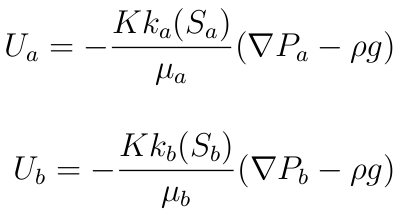State equations :

We assume that the flow  is isothermal, moreover the density and the viscosity are supposed constant.

Continuity equations :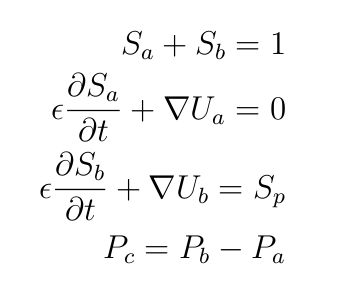# Resolution method

From the previous equations we obtained equation on the pressure :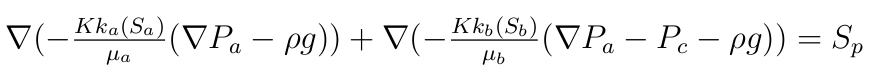The pressure in the phase b is obtained by subtraction.

And we resolved the equation of the saturation of the phase b only :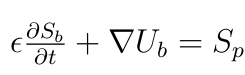The saturation of the phase a is obtained by subtraction.

In the developed two-phase flow  solver we used a method called IMPES (Implicit Pressure Explicit Saturation). In this method the equation on the pressure is resolved in an implicit manner. Moreover the saturation of the liquid phase is resolved explicitly.

Then we resolve the equations of pressure and saturation separately. The positive point of this method is that we gain time of calculation, however the IMPES method could create in certain configurations instabilities during the saturation transport.

# Negative saturation problem

In some cases and due to the instabilities created by the IMPES Method we observe that after certain time of extraction of liquid, the saturation could be negative or superior to one which is incompatible with our definition of the saturation.

Then we added a criteria on the saturation to avoid having negative saturation. The criteria stops the well production if there is a negative saturation in one or several cells of the well.

To resolve saturation superior to one problem, one of the solution  is to decrease gradually the source term (flow rate) when the saturation goes below certain values fixed by the operator. This could help to delay the apparition of saturation superior to one.

# Simulation of different regimes (without capillary pressure)

The aim of this part is to highlight the existence of different regimes that depend on the source term ( flow rate in the well) , on the fluid and the ground properties.

We will launch different simulations for different rate flow values to detect those regimes.

To study the existence of different regime we will plot the saturation for different times and for different flow rates values.

The domain dimension for all the following simulations is 10m*10m.

# Oil production : low flow rate regime

We have a mesh 40*40 cells and the a well of 20cm*50cm.

In the following simulation when the oil saturation goes under the value 0.1 we stop the well production.

we had the following parameters :

phase a :

•  Viscosity $\mu_a=1.17\ kg/(m.s)$
• density $\rho_a=1.85 \ 10^{-5} kg/m^3$

phase b :

•  Viscosity $\mu_b=1 \ 10^{-1} kg/(m.s)$
• density $\rho_b=0.88 \ 10^3 Kg/m^3$

The initial flow rate qwell was fixed to :

•  $qwell=5 \ 10^{-5} m^3/s$

Moreover we consider that we have an isotropic domain and we fixed the permeability to :

•  $K=1 \ 10^{-9}m^2$

Here we can see the representation of the domain :

Well and domain representationThis the initial saturation that  has been taken :

Initial oil staurationSaturation in oil time=63500 sSaturation in oil time=203500 sAs the oil considered in those simulations is very viscous ( $\mu_b=1 \ 10^{-1} kg/(m.s)$ ) this decreases the mobility and it is not possible to pump with high value of the rate flow. Indeed pumping very viscous liquid with high rate flow results in a creation of a "hole in the domain" and the introduction of air in the well which disturb significantly the production. The rate flow adapted to this liquid/configuration should be around $qwell=1 \ 10^{-5} m^3/s$}.

Example of " hole" in the saturation created in the case of $qwell=5 10^{-5} \ m^3/s$ and $K=10^{-10} m^2$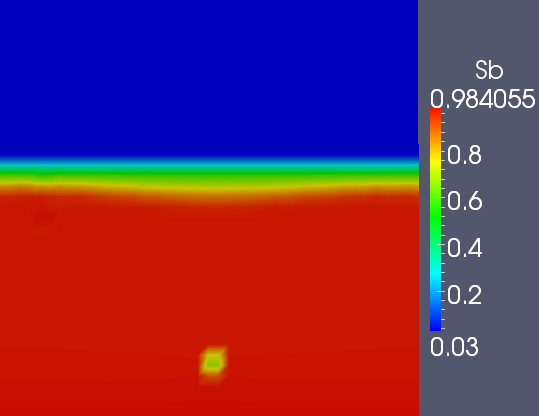Indeed we can see on the previous figure that with very viscous fluid and very low permeability we cannot pump with very high flow rates.

# Water production : high flow rate regime

We have a mesh 120*120 cells and the a well of 50cm*200cm.

We took in this part of fluid that have properties similar to the water properties but less dense than water.

Here we can see the representation of the domain :

Well and domain representationThe initial saturation will have the form of this :

Initial saturation in waterWe implemented a first simulation with the following parameters

phase a :

• Viscosity $\mu_a=1.17 \ kg/(m.s)$
•  density $\rho_a=1.85 \ 10^{-5} kg/m^3$

phase b :

• Viscosity $\mu_b=1 \ 10^{-3} kg/(m.s)$
• density $\rho_b=0.88 \ 10 ^3 Kg/m^3$

The initial flow rate qwell was fixed to :

•  $qwell=1 \ 10^{-2} m^3/s$

Moreover we consider that we have an isotropic domain and we set the permeability to :

•  $K=1 \ 10^{-10}m^2$

In this part when the saturation in water goes below 0.5, the initial flow rate is gradually decreased. Furthermore  when the water saturation goes below the value 0.1 we stop the well production.

Saturation in water time=300 sSaturation in water time=350 sSaturation in water time=650 sSaturation in water time=4000 s# Capillary pressure influence

As it is known, in multiphase flow it could exist a  difference of pressure between phases present in the porous media. The difference of pressure is called the capillary pressure and is due to the existence of surface tension between the phases involved.

When we take into account the capillary pressure, we have a gradient of this pressure added to the conservation equations.

# Capillary pressure modeling

In the literature  exists several empirical formula of capillary pressure present in a porous media.

To understand the effect of the capillary pressure on the saturation, we did our simulation with the following simple formula as a first approach :

$P_c=C(S_e)^{-\alpha}$

where :

•  $P_c$ is the capillary pressure
•  $C$ and $\alpha$ are constant
•  $S_e$ is the effective saturation in liquid.

Se is defined by :• where $S_{min}$ and $S_{max}$ are the minimal and the maximal saturation of the porous media.

# Simulations

We did all the  simulations in this part with the same parameters as in 3.3.1 and by taking into accounting the presence of a capillary pressure with $\alpha=0.2$ and $C=10.10^3 Pa$.

We are going to compare the oil saturation over a vertical line from the domain with capillary pressure and without capillary pressure.

Plot line :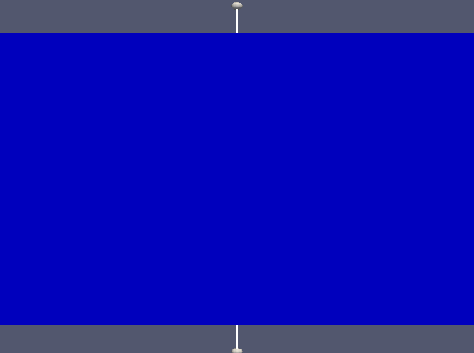# Simulations with capillary pressure and qwell=0

In order to observe the effect of the capillary pressure on the saturation we set the source term equal to 0.

Oil saturation t=0 sOil saturation t=130000 sWe observe that saturation is increased in the boundary region between oil and air. Indeed the water goes up due to the capillary pressure, after certain time the water stop going up due the balance by gravity

# Simulations with capillary pressure with pumping

We compare the influence the case with capillary and the case without capillary pressure.

Saturation without $P_c$, time=100000 sSaturation with $P_c$,  time=100000 sWe observe that the capillary pressure allows the oil to go up and help to the pumping .
Moreover we know that pumping create a lack of oil which increases the influence of the capillary pressure.

# Two phase flow conclusion

We succeeded to implement the solver and resolve problems such as the problem of negative saturation.

However some cases with high  rate flow production are not yet stabilize and leads to a saturation superior to one which causes a divergence of the solver.

The study of viscous flow and in domains with small permeability  has shown that we  have a limit on the rate flow of production. Indeed when this limit is reached a "hole" is created in the liquid saturation field.

# Conclusion

During this project we developed two solvers, one solver in one phase flow and a solver for two phase flow.

The solvers developed take into account the gravity. Moreover as we have seen in the two phase flow, we can have problem of saturation superior to one due to the instabilities created by the IMPES method. One of the solution to delay the apparition of those instabilities is to decrease gradually the rate flow.

In the two phase flow we implemented the capillary pressure with an empirical law. The observations on the capillary pressure were conform to the theory.

In solvers taking into account the gravity, the permeability was not defined as a tensor, because of the need of the inversion of the permeability tensor to put a boundary condition on the pressure gradient on the bottom of the domain. Indeed the operation of tensor inversion is difficult to implement on OpenFoam. Then the permeability was defined as scalar  or as a field of scalar.

Through the different simulations we observed the existence of different regimes, in fact the rate flow or the source term has to be adapted to the fluid and to the porous media properties.

The study of viscous flow in a domain with very small permeability has shown that there is a limit on the maximal flow rate of pumping.

This project will be continued to develop a three phase flow solver and to implement the permeability as a tensor.

# Bibliography

Transfert Milieux Poreux Marc Prat 2006

Petroleum reservoir simulation Khalid AZIZ (PHD) 1979

OpenFoam Jasak

Brooks R.H., Corey A. T., Hydraulic properties of porous media, Hydrology, (1964)